# Heat and Mass Transfer Fundamentals Applications Fourth Edition

• Slides: 48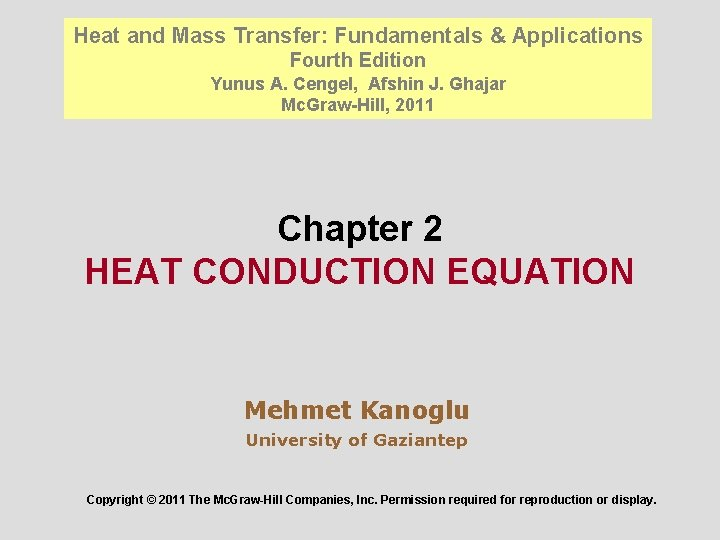Heat and Mass Transfer: Fundamentals & Applications Fourth Edition Yunus A. Cengel, Afshin J. Ghajar Mc. Graw-Hill, 2011 Chapter 2 HEAT CONDUCTION EQUATION Mehmet Kanoglu University of Gaziantep Copyright © 2011 The Mc. Graw-Hill Companies, Inc. Permission required for reproduction or display.Objectives • Understand multidimensionality and time dependence of heat transfer, and the conditions under which a heat transfer problem can be approximated as being one-dimensional. • Obtain the differential equation of heat conduction in various coordinate systems, and simplify it for steady one-dimensional case. • Identify thermal conditions on surfaces, and express them mathematically as boundary and initial conditions. • Solve one-dimensional heat conduction problems and obtain the temperature distributions within a medium and the heat flux. • Analyze one-dimensional heat conduction in solids that involve heat generation. • Evaluate heat conduction in solids with temperature-dependent thermal conductivity. 2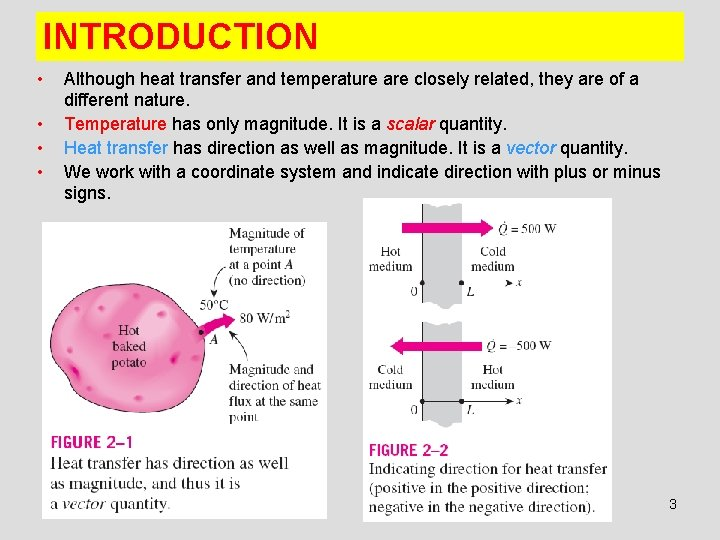INTRODUCTION • • Although heat transfer and temperature are closely related, they are of a different nature. Temperature has only magnitude. It is a scalar quantity. Heat transfer has direction as well as magnitude. It is a vector quantity. We work with a coordinate system and indicate direction with plus or minus signs. 3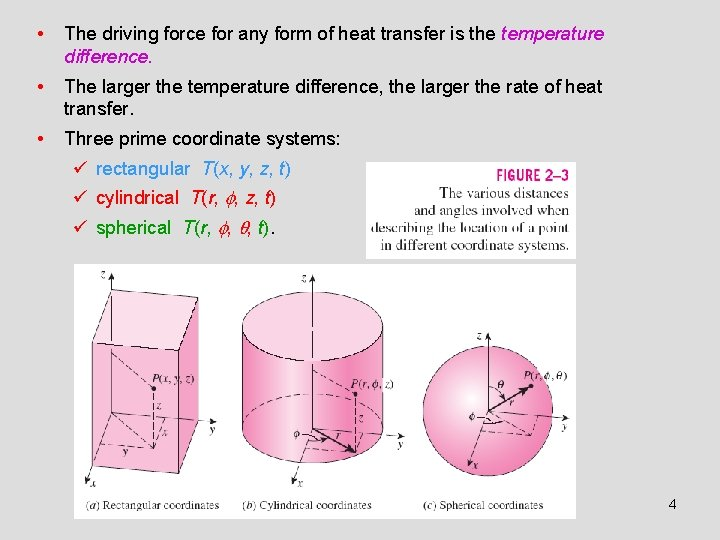• The driving force for any form of heat transfer is the temperature difference. • The larger the temperature difference, the larger the rate of heat transfer. • Three prime coordinate systems: ü rectangular T(x, y, z, t) ü cylindrical T(r, , z, t) ü spherical T(r, , , t). 4Steady versus Transient Heat Transfer • Steady implies no change with time at any point within the medium • Transient implies variation with time or time dependence • In the special case of variation with time but not with position, the temperature of the medium changes uniformly with time. Such heat transfer systems are called lumped systems. 5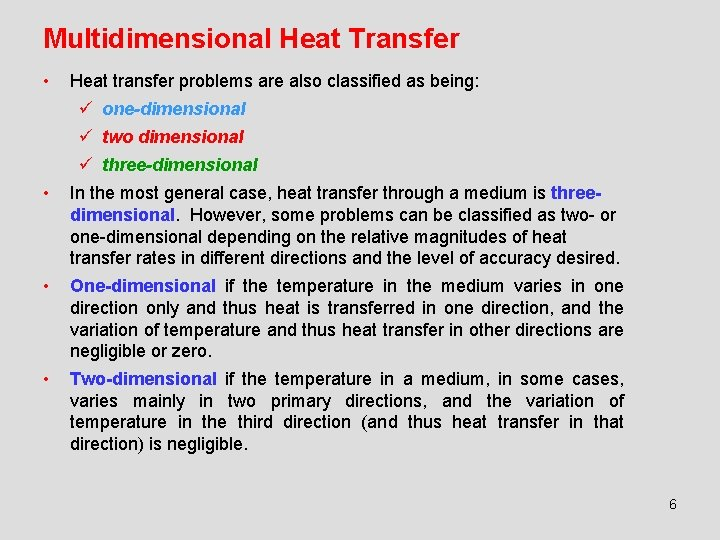Multidimensional Heat Transfer • Heat transfer problems are also classified as being: ü one-dimensional ü two dimensional ü three-dimensional • In the most general case, heat transfer through a medium is threedimensional. However, some problems can be classified as two- or one-dimensional depending on the relative magnitudes of heat transfer rates in different directions and the level of accuracy desired. • One-dimensional if the temperature in the medium varies in one direction only and thus heat is transferred in one direction, and the variation of temperature and thus heat transfer in other directions are negligible or zero. • Two-dimensional if the temperature in a medium, in some cases, varies mainly in two primary directions, and the variation of temperature in the third direction (and thus heat transfer in that direction) is negligible. 67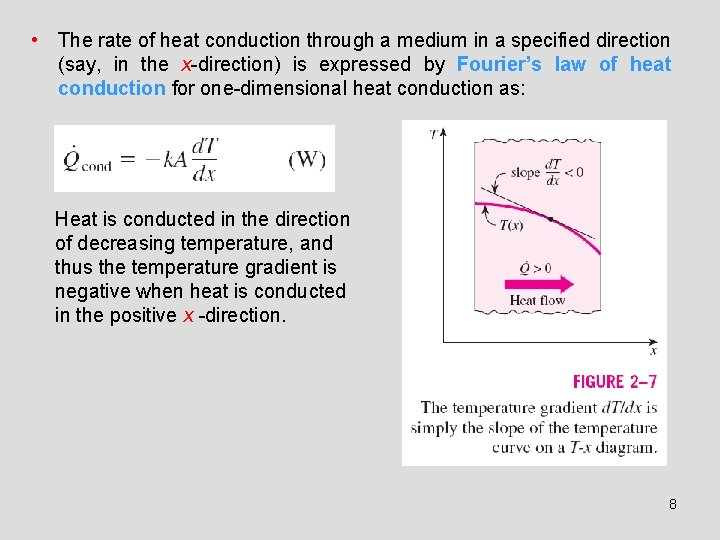• The rate of heat conduction through a medium in a specified direction (say, in the x-direction) is expressed by Fourier’s law of heat conduction for one-dimensional heat conduction as: Heat is conducted in the direction of decreasing temperature, and thus the temperature gradient is negative when heat is conducted in the positive x -direction. 8• The heat flux vector at a point P on the surface of the figure must be perpendicular to the surface, and it must point in the direction of decreasing temperature • If n is the normal of the isothermal surface at point P, the rate of heat conduction at that point can be expressed by Fourier’s law as 9• • Examples: ü electrical energy being converted to heat at a rate of I 2 R, ü fuel elements of nuclear reactors, ü exothermic chemical reactions. Heat generation is a volumetric phenomenon. The rate of heat generation units : W/m 3 or Btu/h·ft 3. The rate of heat generation in a medium may vary with time as well as position within the medium. Heat Generation 10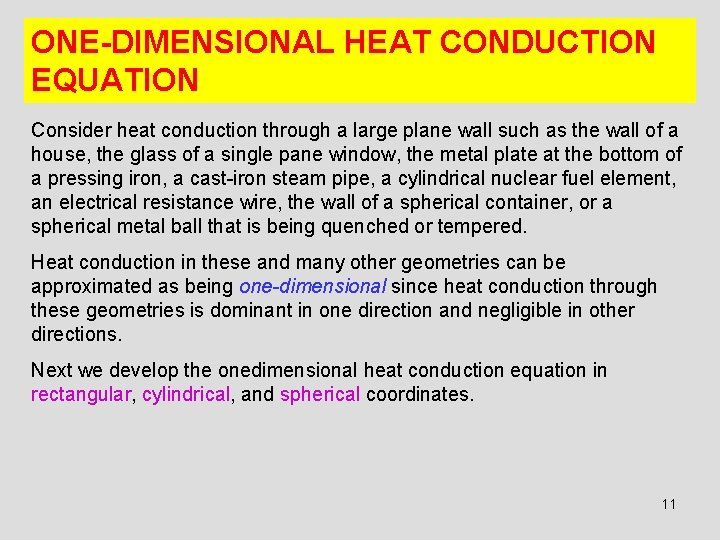ONE-DIMENSIONAL HEAT CONDUCTION EQUATION Consider heat conduction through a large plane wall such as the wall of a house, the glass of a single pane window, the metal plate at the bottom of a pressing iron, a cast-iron steam pipe, a cylindrical nuclear fuel element, an electrical resistance wire, the wall of a spherical container, or a spherical metal ball that is being quenched or tempered. Heat conduction in these and many other geometries can be approximated as being one-dimensional since heat conduction through these geometries is dominant in one direction and negligible in other directions. Next we develop the onedimensional heat conduction equation in rectangular, cylindrical, and spherical coordinates. 11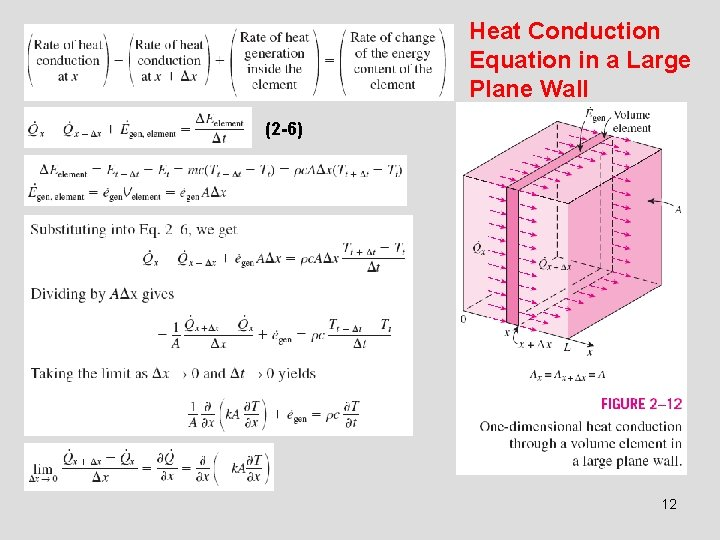Heat Conduction Equation in a Large Plane Wall (2 -6) 12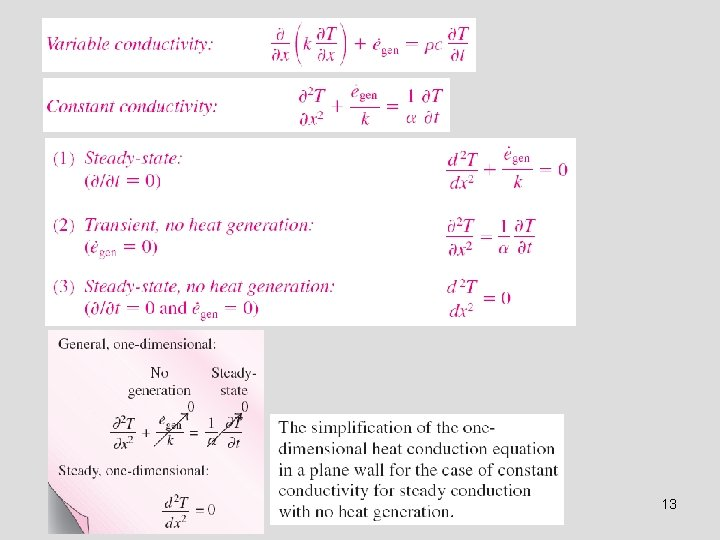13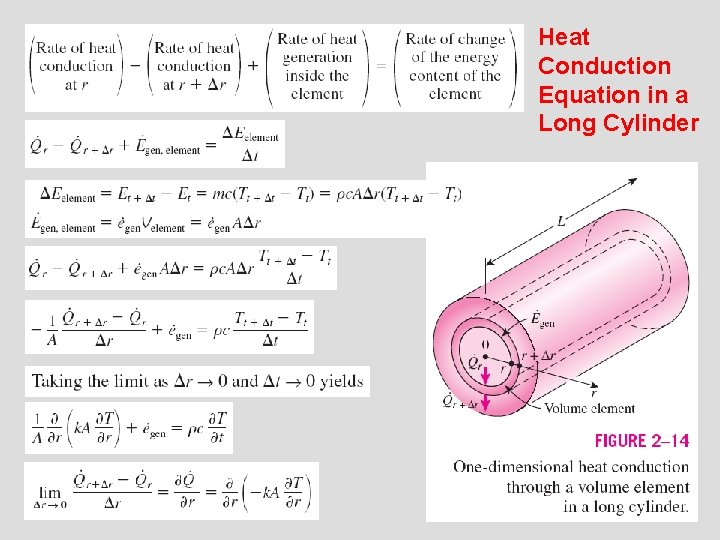Heat Conduction Equation in a Long Cylinder 1415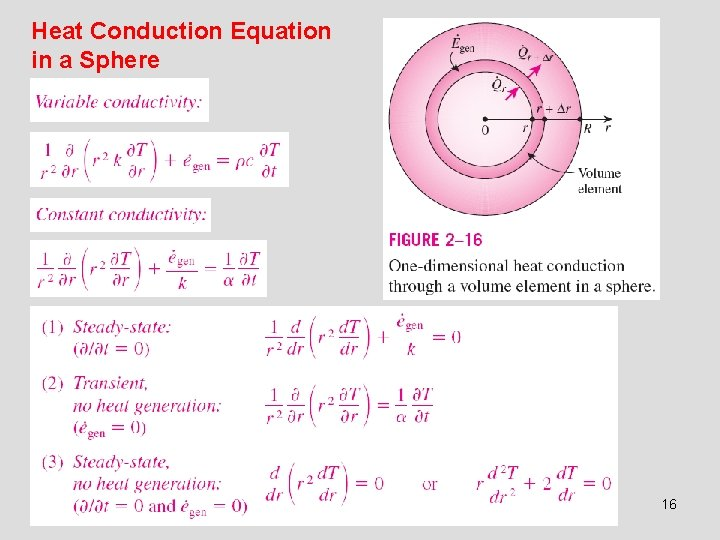Heat Conduction Equation in a Sphere 16Combined One-Dimensional Heat Conduction Equation An examination of the one-dimensional transient heat conduction equations for the plane wall, cylinder, and sphere reveals that all three equations can be expressed in a compact form as n = 0 for a plane wall n = 1 for a cylinder n = 2 for a sphere In the case of a plane wall, it is customary to replace the variable r by x. This equation can be simplified for steady-state or no heat generation cases as described before. 17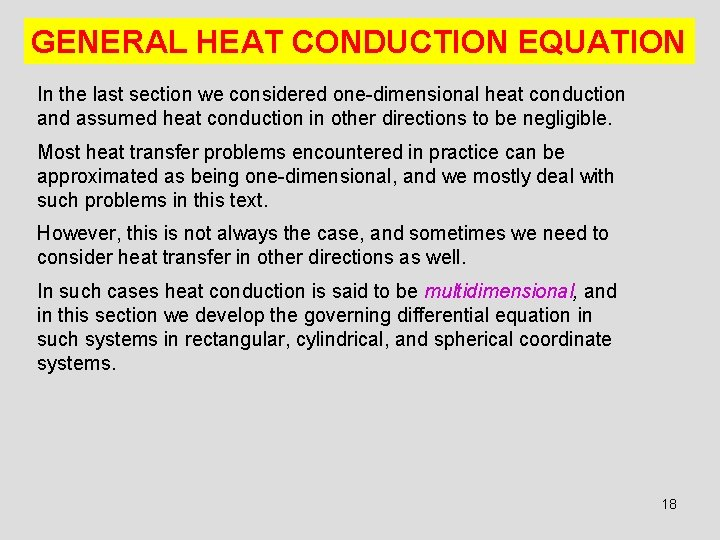GENERAL HEAT CONDUCTION EQUATION In the last section we considered one-dimensional heat conduction and assumed heat conduction in other directions to be negligible. Most heat transfer problems encountered in practice can be approximated as being one-dimensional, and we mostly deal with such problems in this text. However, this is not always the case, and sometimes we need to consider heat transfer in other directions as well. In such cases heat conduction is said to be multidimensional, and in this section we develop the governing differential equation in such systems in rectangular, cylindrical, and spherical coordinate systems. 18Rectangular Coordinates 1920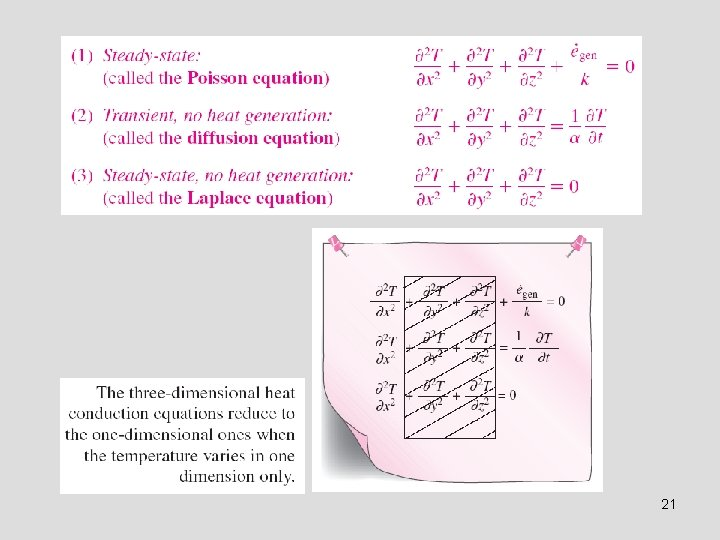21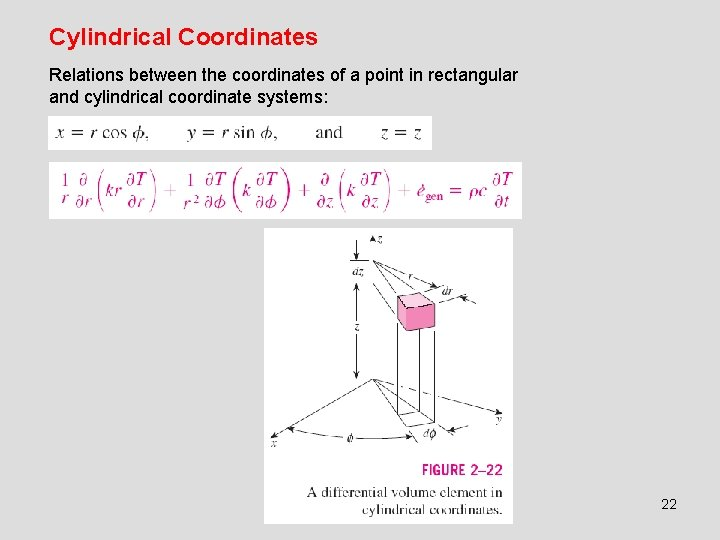Cylindrical Coordinates Relations between the coordinates of a point in rectangular and cylindrical coordinate systems: 22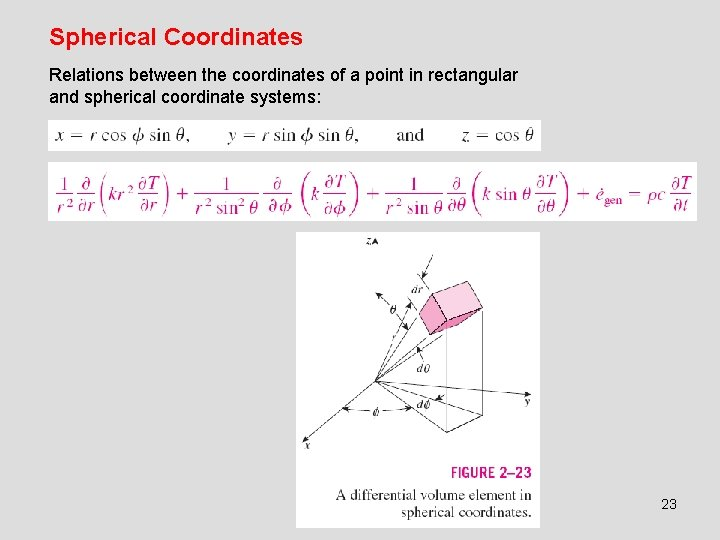Spherical Coordinates Relations between the coordinates of a point in rectangular and spherical coordinate systems: 23BOUNDARY AND INITIAL CONDITIONS The description of a heat transfer problem in a medium is not complete without a full description of thermal conditions at the bounding surfaces of the medium. Boundary conditions: The mathematical expressions of thermal conditions at the boundaries. The temperature at any point on the wall at a specified time depends on the condition of the geometry at the beginning of the heat conduction process. Such a condition, which is usually specified at time t = 0, is called the initial condition, which is a mathematical expression for the temperature distribution of the medium initially. 24Boundary Conditions • Specified Temperature Boundary Condition • Specified Heat Flux Boundary Condition • Convection Boundary Condition • Radiation Boundary Condition • Interface Boundary Conditions • Generalized Boundary Conditions 25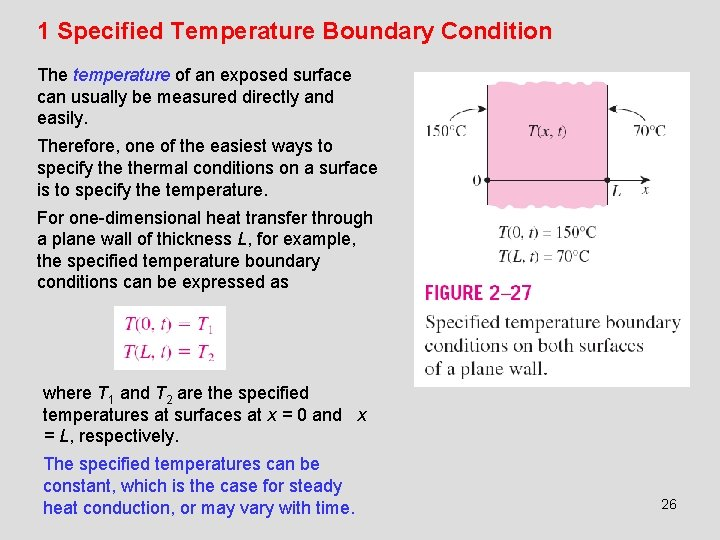1 Specified Temperature Boundary Condition The temperature of an exposed surface can usually be measured directly and easily. Therefore, one of the easiest ways to specify thermal conditions on a surface is to specify the temperature. For one-dimensional heat transfer through a plane wall of thickness L, for example, the specified temperature boundary conditions can be expressed as where T 1 and T 2 are the specified temperatures at surfaces at x = 0 and x = L, respectively. The specified temperatures can be constant, which is the case for steady heat conduction, or may vary with time. 26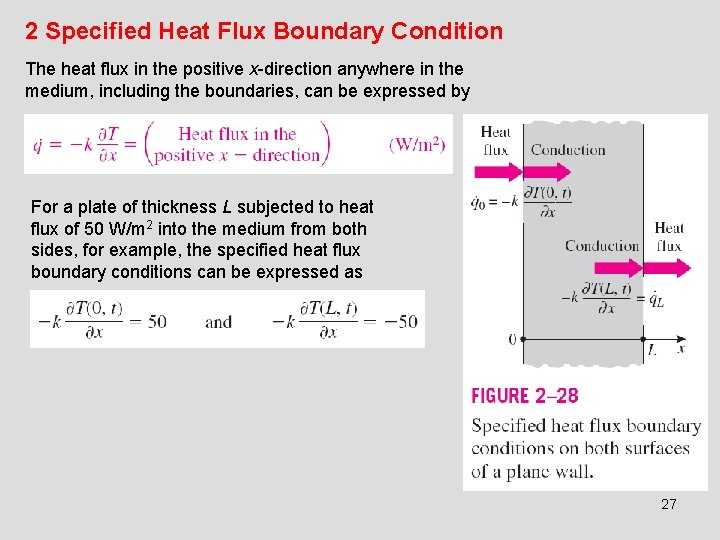2 Specified Heat Flux Boundary Condition The heat flux in the positive x-direction anywhere in the medium, including the boundaries, can be expressed by For a plate of thickness L subjected to heat flux of 50 W/m 2 into the medium from both sides, for example, the specified heat flux boundary conditions can be expressed as 27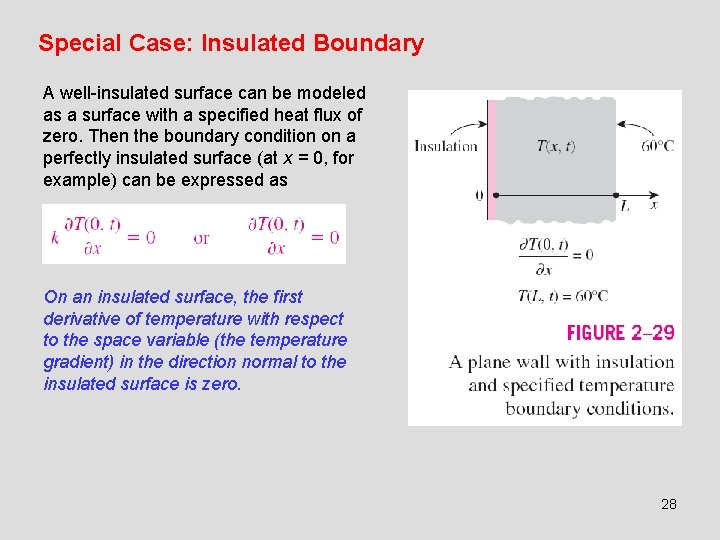Special Case: Insulated Boundary A well-insulated surface can be modeled as a surface with a specified heat flux of zero. Then the boundary condition on a perfectly insulated surface (at x = 0, for example) can be expressed as On an insulated surface, the first derivative of temperature with respect to the space variable (the temperature gradient) in the direction normal to the insulated surface is zero. 28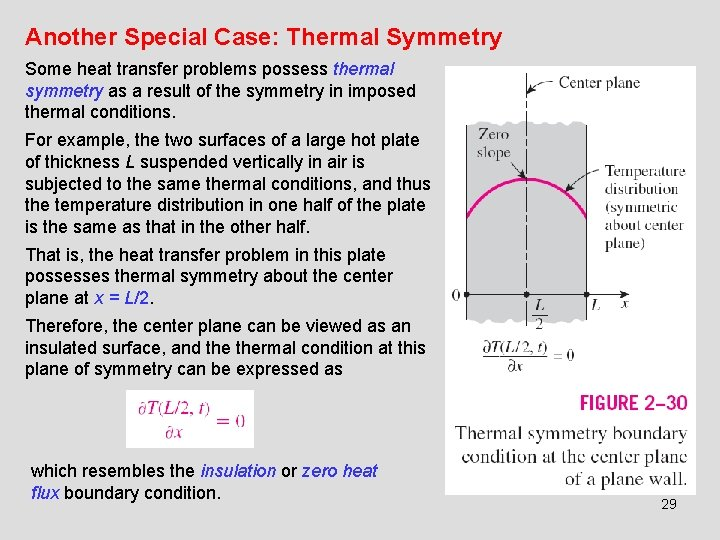Another Special Case: Thermal Symmetry Some heat transfer problems possess thermal symmetry as a result of the symmetry in imposed thermal conditions. For example, the two surfaces of a large hot plate of thickness L suspended vertically in air is subjected to the same thermal conditions, and thus the temperature distribution in one half of the plate is the same as that in the other half. That is, the heat transfer problem in this plate possesses thermal symmetry about the center plane at x = L/2. Therefore, the center plane can be viewed as an insulated surface, and thermal condition at this plane of symmetry can be expressed as which resembles the insulation or zero heat flux boundary condition. 293 Convection Boundary Condition For one-dimensional heat transfer in the x-direction in a plate of thickness L, the convection boundary conditions on both surfaces: 30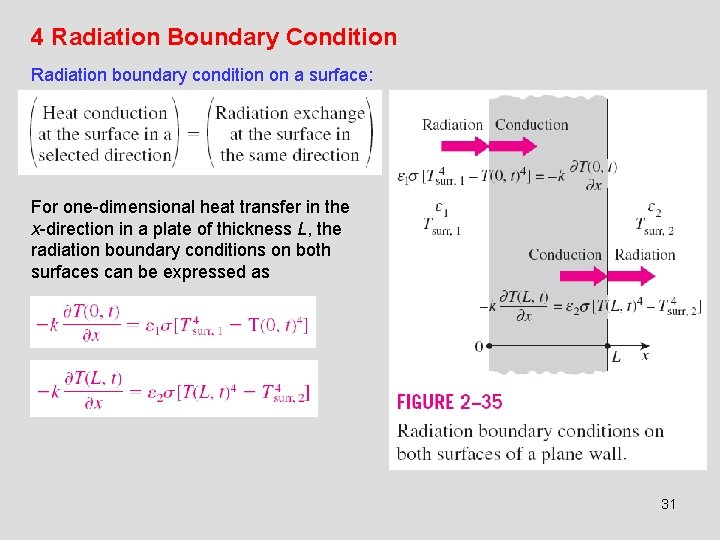4 Radiation Boundary Condition Radiation boundary condition on a surface: For one-dimensional heat transfer in the x-direction in a plate of thickness L, the radiation boundary conditions on both surfaces can be expressed as 315 Interface Boundary Conditions The boundary conditions at an interface are based on the requirements that (1) two bodies in contact must have the same temperature at the area of contact and (2) an interface (which is a surface) cannot store any energy, and thus the heat flux on the two sides of an interface must be the same. The boundary conditions at the interface of two bodies A and B in perfect contact at x = x 0 can be expressed as 32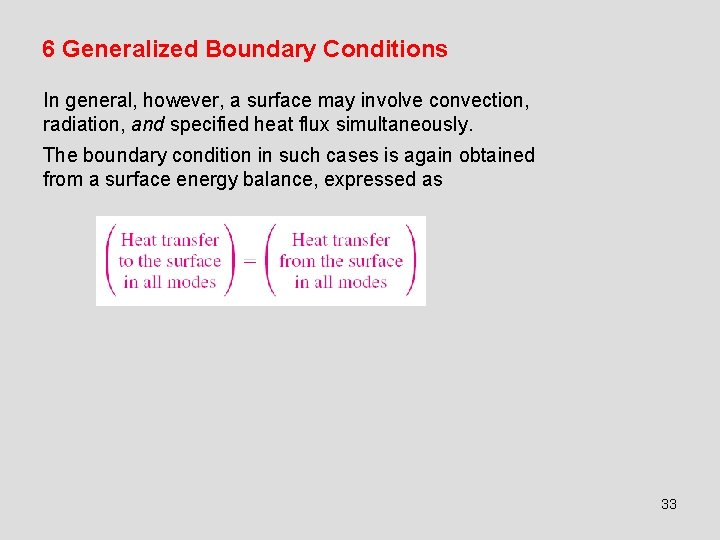6 Generalized Boundary Conditions In general, however, a surface may involve convection, radiation, and specified heat flux simultaneously. The boundary condition in such cases is again obtained from a surface energy balance, expressed as 33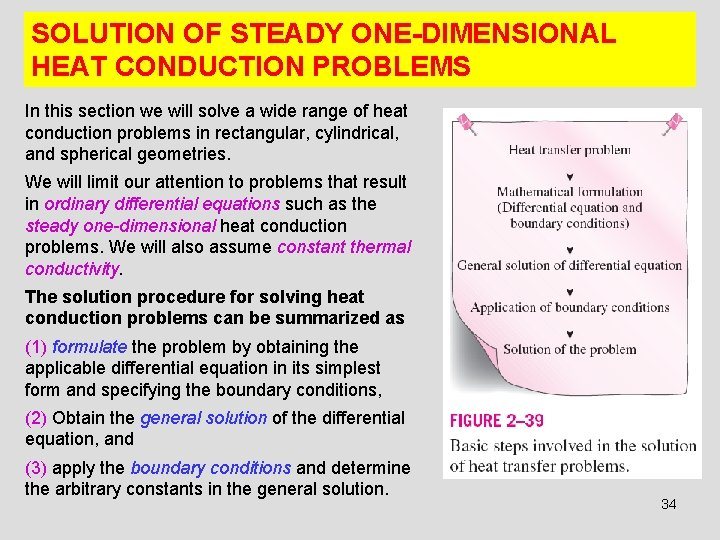SOLUTION OF STEADY ONE-DIMENSIONAL HEAT CONDUCTION PROBLEMS In this section we will solve a wide range of heat conduction problems in rectangular, cylindrical, and spherical geometries. We will limit our attention to problems that result in ordinary differential equations such as the steady one-dimensional heat conduction problems. We will also assume constant thermal conductivity. The solution procedure for solving heat conduction problems can be summarized as (1) formulate the problem by obtaining the applicable differential equation in its simplest form and specifying the boundary conditions, (2) Obtain the general solution of the differential equation, and (3) apply the boundary conditions and determine the arbitrary constants in the general solution. 34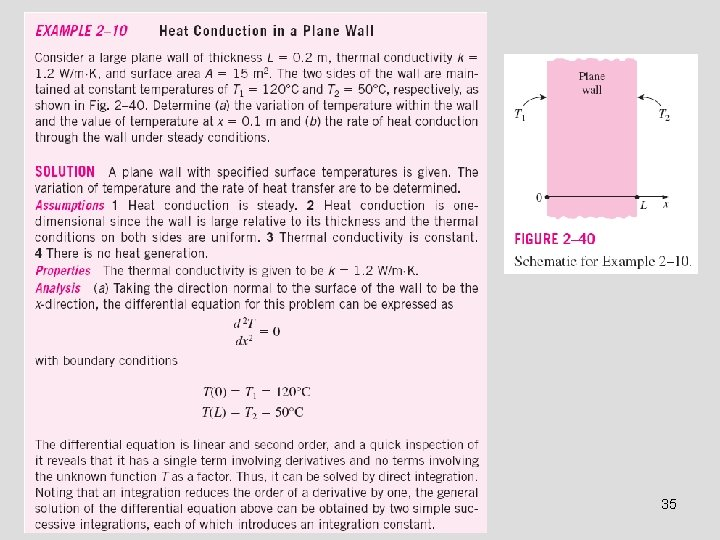353637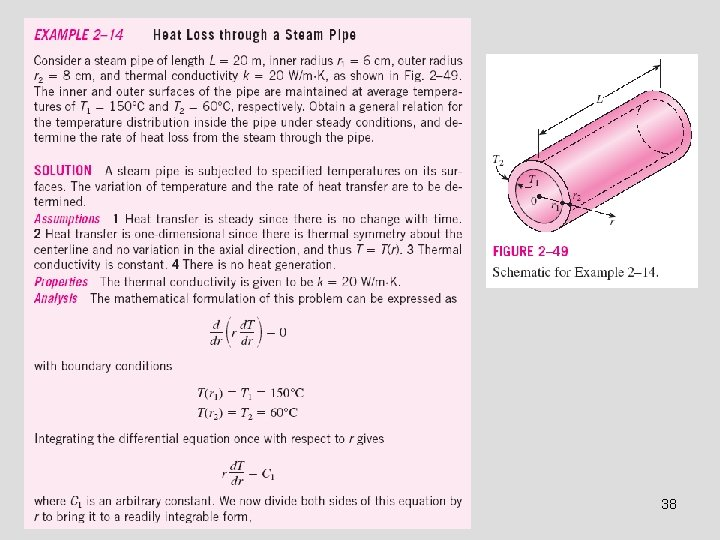38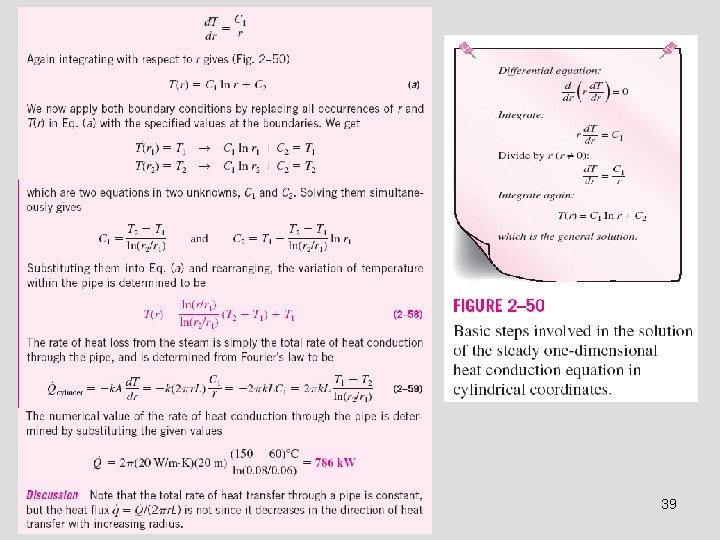39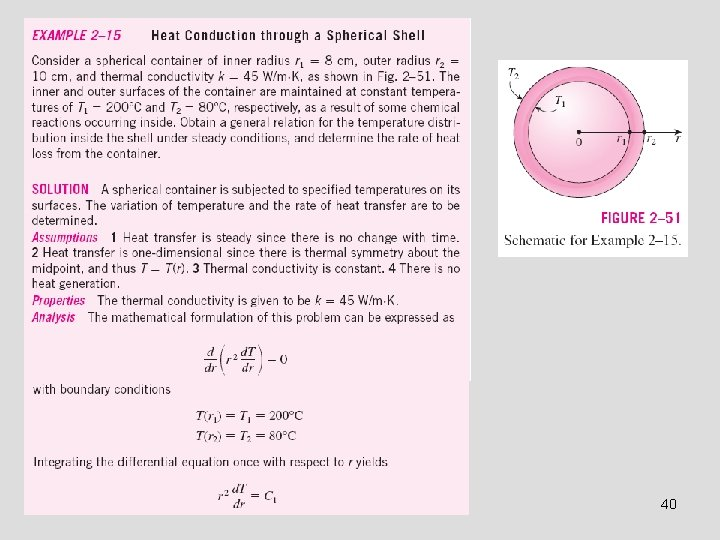40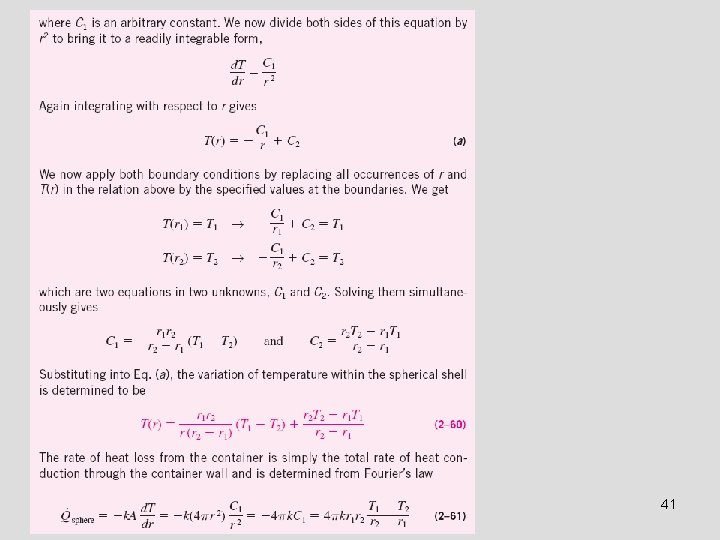41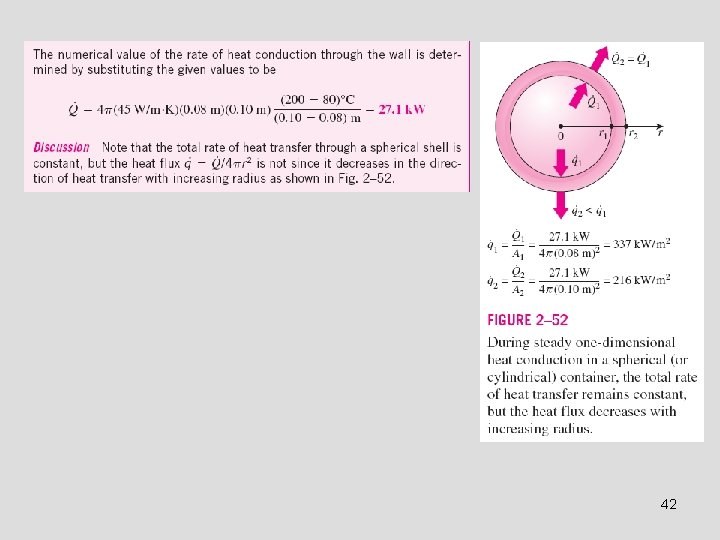42HEAT GENERATION IN A SOLID Many practical heat transfer applications involve the conversion of some form of energy into thermal energy in the medium. Such mediums are said to involve internal heat generation, which manifests itself as a rise in temperature throughout the medium. Some examples of heat generation are - resistance heating in wires, - exothermic chemical reactions in a solid, and - nuclear reactions in nuclear fuel rods where electrical, chemical, and nuclear energies are converted to heat, respectively. Heat generation in an electrical wire of outer radius ro and length L can be expressed as 43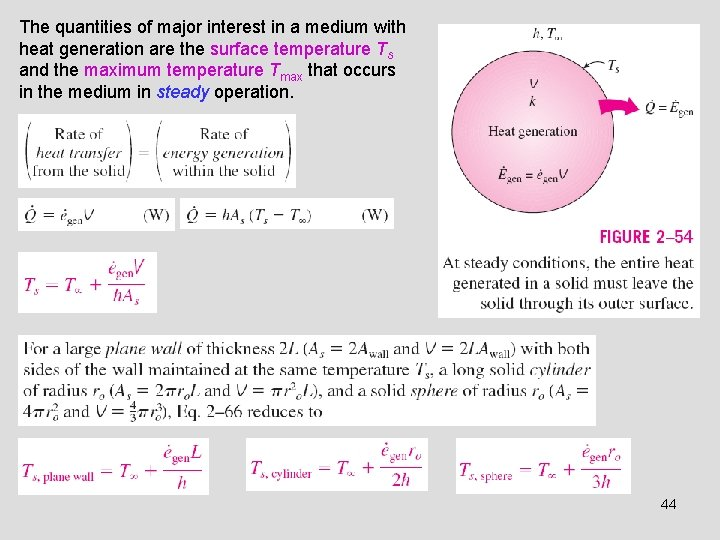The quantities of major interest in a medium with heat generation are the surface temperature Ts and the maximum temperature Tmax that occurs in the medium in steady operation. 4445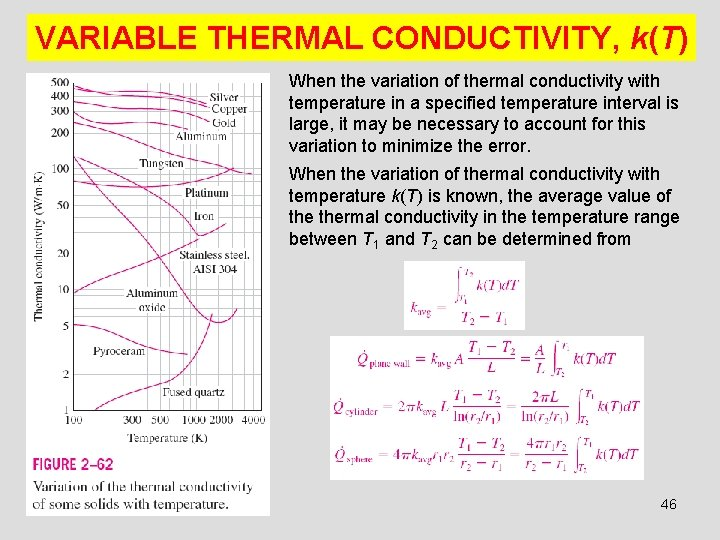VARIABLE THERMAL CONDUCTIVITY, k(T) When the variation of thermal conductivity with temperature in a specified temperature interval is large, it may be necessary to account for this variation to minimize the error. When the variation of thermal conductivity with temperature k(T) is known, the average value of thermal conductivity in the temperature range between T 1 and T 2 can be determined from 46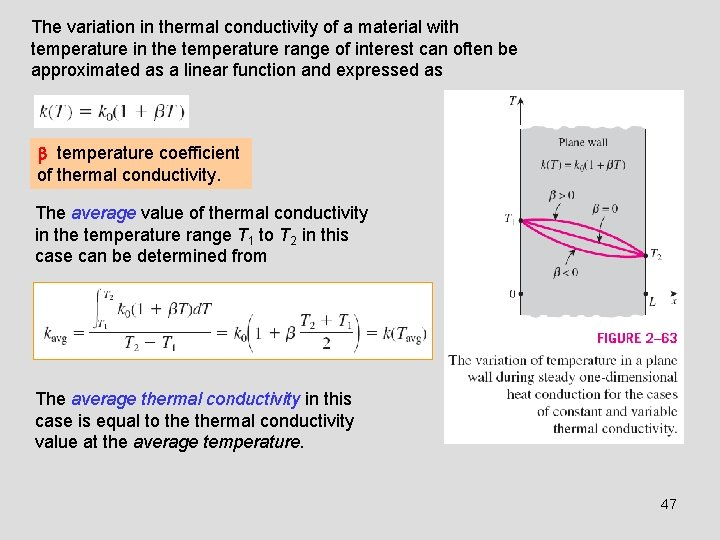The variation in thermal conductivity of a material with temperature in the temperature range of interest can often be approximated as a linear function and expressed as temperature coefficient of thermal conductivity. The average value of thermal conductivity in the temperature range T 1 to T 2 in this case can be determined from The average thermal conductivity in this case is equal to thermal conductivity value at the average temperature. 47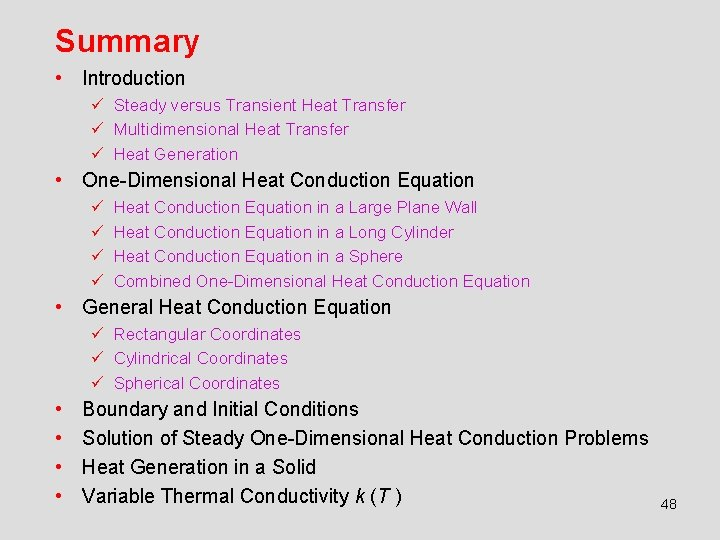Summary • Introduction ü Steady versus Transient Heat Transfer ü Multidimensional Heat Transfer ü Heat Generation • One-Dimensional Heat Conduction Equation ü ü Heat Conduction Equation in a Large Plane Wall Heat Conduction Equation in a Long Cylinder Heat Conduction Equation in a Sphere Combined One-Dimensional Heat Conduction Equation • General Heat Conduction Equation ü Rectangular Coordinates ü Cylindrical Coordinates ü Spherical Coordinates • • Boundary and Initial Conditions Solution of Steady One-Dimensional Heat Conduction Problems Heat Generation in a Solid Variable Thermal Conductivity k (T ) 48